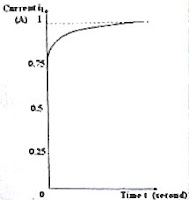## Pages

`“Life is like riding a bicycle.  To keep your balance you must keep moving.”–Albert Einstein`

## Monday, December 24, 2007

### Answer to AP Physics Free Response Question on Inductance

A free response question involving inductance was posted on 23rd December 2007 for your practice. This was the question:In the circuit shown in the figure, the battery and the inductor have negligible resistance. The currents through R1, R2 and R3 are i1, i2 and i3 respectively. The switch S is closed at t = 0.

(a) Calculate the currents i1, i2 and i3 immediately after closing the switch S (when t = 0).

(b) Calculate the currents i1, i2 and i3 when steady state is reached.

(c) Show the nature of variation of the current through the battery graphically, with time ‘t’ on the X-axis and the current (i1) on the Y-axis.

(d) What are the values of the slopes of the above graph at t = 0 and at t = ∞.

(e) The switch S is opened and the resistance R3 is disconnected. If the switch S is now closed, what time is required for the current to attain 63.2% of the final steady value?

As promised, I give below the answer:

(a) Since the parallel branch containing R3 does not contain any inductance, the current through that branch (i3) rises to the maximum possible value in this condition immediately on closing the switch S. The same current now flows through R1 since the initial current i2 ( at t = 0) through the branch containing the inductance L and the resistance R2 is zero. [Note that in an LR circuit, the current I at the instant ‘t’ is given by I = I0(1 e–Rt/L) where I0 is the final steady current]

Therefore, when t = 0, i1 = i3 = (12 V)/ (8 Ω + 8 Ω ) = 0.75 A and i2 = 0

(b) Steady state is reached when t = ∞. In this condition, the current is controlled by the resistances only [since dI/dt is zero and no opposing induced voltage L(dI/dt) is developed across the inductance]. The circuit then behaves as made of the parallel combination of R2 and R3 (having effective value of 4 Ω) in series with the battery and the resistance R1. The effective resistance of the circuit is (8 + 4) Ω = 12 Ω and the current delivered by the battery (i1) is (12 V)/ (12 Ω) = 1 A.

Since this current is equally divided between the two parallel branches, current through each branch is 0.5 A. So, i2 = i3 = 0.5 A.(c) The variation of the current i1 with time t is shown in the adjoining graph. [The current grows exponentially in the inductive branch and this is why the current delivered by the battery finally rises to the steady value of 1 A as shown].

(d) The slope of the graph when t = o is infinity since the current grows abruptly to 0.75 A because of the purely resistive parallel branch containing R3. The slope when t = ∞ is zero since the current has settled to the final steady value.

(e) When R3 is disconnected, We have a series LR circuit with L = 2 H and R = R1 + R2 = 16 Ω. The time constant of the circuit is L/R = 2/16 = 0.125 s. The current grows to 63.2 % of the final steady current within this time.

[Time constant is L/R which is the time required for the current to become (1 – 1/e) times the final steady current. This is obtained by putting t = L/R in the equation,

I = I0(1 e–Rt/L)].

Merry Christmas!